# Primary 4 CA1 Practice #3

Time elapsed:
Q1. Use a protractor to measure ∠m.     [1 mark]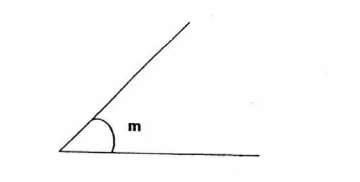Q2. Find the product of 134 and 35.     [1 mark]
Q3. Yvvone want to shade 57 of the figure below. How many more squares must she shade?     [1 mark]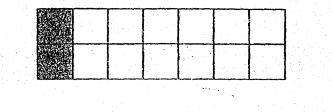Q4. William is at point X facing north-west. He makes a 90° clockwise turn. Then he makes a _____ in the anti-clockwise direction to face south-east.     [1 mark]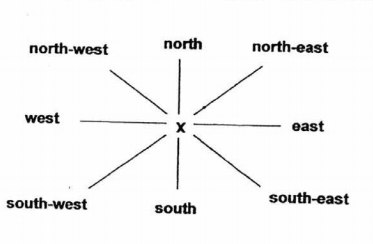Q5. Which of the following is not an equivalent fraction of 13?     [1 mark]
Q6. Round off 12 946 to the nearest ten.     [1 mark]
Q7. When a number is divided by 6, the quotient is 7 and the remainder is 4. What is the number?     [2 marks]
Q8. Which of the following are factors of 36?     [1 mark]
Q9. Use the given protractor to find ∠WYZ.     [1 mark]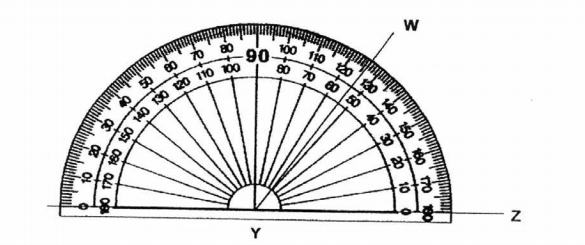Q10. Divide 9237 by 6. What is the remainder?     [1 mark]
Q11. Xena bought 36 sets of stickers. Each set contains 78 stickers. How many stickers did Xena buy? Round your answer to the nearest ten.     [1 mark]
Q12. What is the sum of the common factors of 16 and 24?     [1 mark]
Q13. Kevin bought a toy train that cost \$5.70. He paid the exact amount using some twenty-cent and fifty cent coins. How many twenty-cent and fifty-cent coins did he use to pay for the toy train?     [1 mark]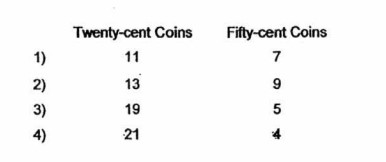Q14. Which of the following is a factor of 56?     [1 mark]
Q15. Meng is facing the library. Where will he be facing if he makes a 34 - turn in the clockwise direction?     [1 mark]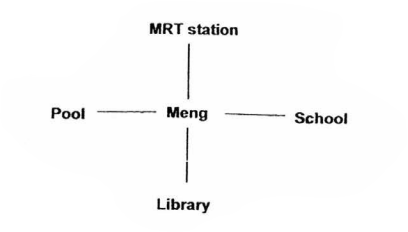Q16. Which of the following statements is incorrect?     [1 mark]
Q17. What is 7595 when rounded to the nearest hundred?     [1 mark]
Q18. Round 29 105 to the nearest ten.     [1 mark]
Q19. 1304 x 7 = ____.     [1 mark]
Q20. Which of the following numbers is the smallest number? 18 369, 18 639, 18 693, 18 936     [1 mark]